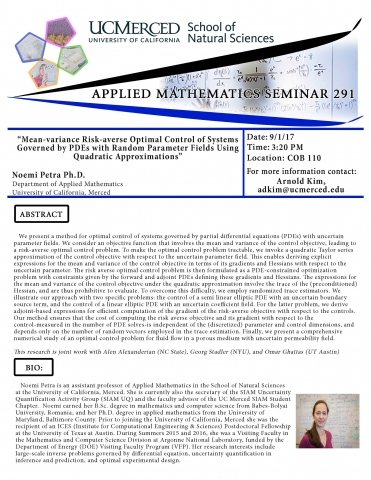# Applied Mathematics Seminar 291 (9/1/17)

September 1, 2017 - 10:30pm## Noemi Petra Ph.D., University of California, Merced

Abstract

We present a method for optimal control of systems governed by partial differential equations (PDEs) with uncertain parameter fields. We consider an objective function that involves the mean and variance of the control objective, leading to a risk-averse optimal control problem. To make the optimal control problem tractable, we invoke a quadratic Taylor series approximation of the control objective with respect to the uncertain parameter field. This enables deriving explicit expressions for the mean and variance of the control objective in terms of its gradients and Hessians with respect to the uncertain parameter. The risk averse optimal control problem is then formulated as a PDE-constrained optimization problem with constraints given by the forward and adjoint PDEs defining these gradients and Hessians. The expressions for the mean and variance of the control objective under the quadratic approximation involve the trace of the (preconditioned) Hessian, and are thus prohibitive to evaluate. To overcome this difficulty, we employ randomized trace estimators. We illustrate our approach with two specific problems: the control of a semi linear elliptic PDE with an uncertain boundary source term, and the control of a linear elliptic PDE with an uncertain coefficient field. For the latter problem, we derive adjoint-based expressions for efficient computation of the gradient of the risk-averse objective with respect to the controls. Our method ensures that the cost of computing the risk averse objective and its gradient with respect to the control-measured in the number of PDE solves-is independent of the (discretized) parameter and control dimensions, and depends only on the number of random vectors employed in the trace estimation. Finally, we present a comprehensive numerical study of an optimal control problem for fluid flow in a porous medium with uncertain permeability field.

Arnold Kim
Professor